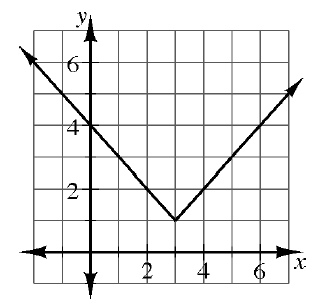### Home > INT3 > Chapter Ch3 > Lesson 3.1.3 > Problem3-50

3-50.

Examine the graph of $f ( x ) = | x - 3 | + 1$ at right. Explain how you can use the graph to determine the values below. Homework Help ✎1. $f(3)$

What $y$-value corresponds with $x=3$?

$1$

1. $f(0)$

$4$

1. $f(4)$

Refer to parts (a) and (b).

1. $f(−1)$

Refer to parts (a) and (b).